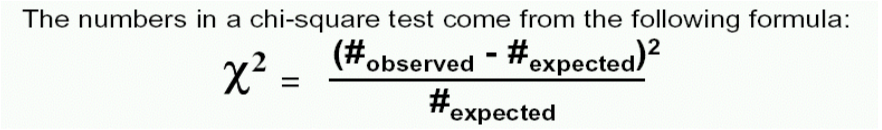Chi Square

Chi-Square- A Chi-square’s probability density curve is an asymmetric curve extending over the positive side of the line and having a long right tail. The form of the curve depends on the value of the degrees of freedom.

Types of Chi-Square Analysis

Chi-square Test for Association is a (non-parametric, therefore can be used for nominal data) test of statistical significance widely used bivariate tabular association analysis.

Normally, the hypothesis is whether or not two different populations are different enough in some characteristic or aspect of their behavior based on two random samples. This test procedure is also known as the Pearson chi-square test. Chi-square Goodness-of-fit Test is used to test if an observed distribution conforms to any particular distribution. Calculation of this goodness of fit test is by comparison of observed data with data expected based on the particular distribution.When to apply a Chi-Squared Test
The chi-Squared test is used to determine if there is a statistically significant difference in the proportions for different groups. To accomplish this, it breaks all outcomes into groups.
What the Chi-Squared Test does
• It starts by determining how many defects, for example, would be “expected” in each group involved.
• It does this by assuming that all groups have the same defect rate (which Minitab approximates from the data provided).
• Minitab then compares the expected counts with what was actually observed.
• If the numbers are different by a large enough amount, Chi-Square determines that the groups do not have the same proportion.
Chi-Square Requirements
• Data is typically attribute (discrete). At the very least, all data must be able to be categorized as being in some category or another).
•
• Expected cell counts should not below (definitely not less than 1 and preferably not less than 5) as this could lead to a false positive indication that there is a difference when, in fact, none exists.
Chi-Square Hypotheses
Ho: The null hypotheses (P-Value > 0.05) means the populations have the same proportions.
Ha: The alternate hypotheses (P-Value <= 0.05) means the populations do NOT have the same proportions.

Test Your Six Sigma Black Belt Skills By Taking Our Practice Tests on This Link

Become Vskills Certified Six Sigma Black Belt Professional. Learn the module “Definition and Functions of Six Sigma”. Try the free practice test!

Apply for Six Sigma Black Belt Certification Now!!

http://www.vskills.in/certification/Certified-Six-Sigma-Black-Belt-Professional